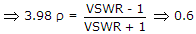# Electronics and Communication Engineering - Electromagnetic Field Theory - Discussion

4.

In a line VSWR of a load is 6 dB. The reflection coefficient will be

 [A]. 0.033 [B]. 0.33 [C]. 0.66 [D]. 3.3

Answer: Option C

Explanation:

VSWR = 100.6Amod Kumar Jha said: (May 27, 2017) It should be 0.33. If voltage or magnitude express in db then the farmula is 20log(v). And anything related to power is only written in 10log(p), it is the actual meaning of decimal means 10. We can remember it by viewing p=v^2/r. Since square term come in the farmula so that 2 goes into multiplication with 10 equal 20, while expressing in log. I hope now it clear to everyone.

#### Post your comments here:

Name *:

Email   : (optional)

» Your comments will be displayed only after manual approval.

#### Current Affairs 2021

Interview Questions and Answers